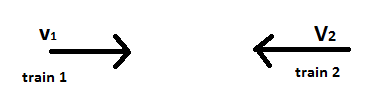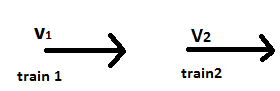×#### Thank you for registering.

One of our academic counsellors will contact you within 1 working day.

Click to Chat

1800-1023-196

+91-120-4616500

CART 0

• 0

MY CART (5)

Use Coupon: CART20 and get 20% off on all online Study Material

ITEM
DETAILS
MRP
DISCOUNT
FINAL PRICE
Total Price: Rs.

There are no items in this cart.
Continue Shopping```
2 identical trains take 3 sec to pass 1 another when going in the opposite direction but only 2.5 sec if speed of 1 is increased by 50%.The time one would take to pass the other when going in the same direction at their original speed is?

```
8 years ago

```							let train A is moving along positie x axis with vel = +V
train B along negative x axis = -U
vel of train A with respect B is = V-(-U) = V+U
let S be distance
S=(V+U)3
S=(V + U + U/2)2.5
=> S= (V+3/2U)2.5
get v and u in terms of S
then apply S=(V-U)t    (now V along and u in same direction v=V-U)
and find t
```
8 years ago
```							working in ref frame attached to 2,velocity of 1 wrt 2= v1+v2
let length of train be x metres each.
therefore time taken to cross each other= 2x/v1+v2=3
=> 2x= 3v1+3v2----------------------------------------------(1)
Case 2:
velocity of 1= v1+50v1/100=3v1/2
now, time taken to cross each other=      2x                                                             -------------- = 2.5
3v1/2- v2
=> 2x= 2.5v2+ 7.5v1/2---------------(2)
equating (1) and (2)
3v1+3v2= 2.5v2+7.5v1/2
=> 1.5v1/2= v2--------------------------------(3)
now if they move in same direction:velocity of 1 wrt 2= v1-v2
time taken to cross each other= 2x/v1-v2
= 2x/v1-0.75v1 (from 3)
= 2x/0.25v1
= 3v1+3 v2/ 0.25v1 (from 1)
= 3v1+ 0.75v1/0.25v1 (from 3)
= 3.75v1/0.25v1
= 15 sec
all the best!
```
7 years ago
Think You Can Provide A Better Answer ?

## Other Related Questions on General Physics

View all Questions »### Course Features

• 731 Video Lectures
• Revision Notes
• Previous Year Papers
• Mind Map
• Study Planner
• NCERT Solutions
• Discussion Forum
• Test paper with Video Solution### Course Features

• 18 Video Lectures
• Revision Notes
• Test paper with Video Solution
• Mind Map
• Study Planner
• NCERT Solutions
• Discussion Forum
• Previous Year Exam Questions